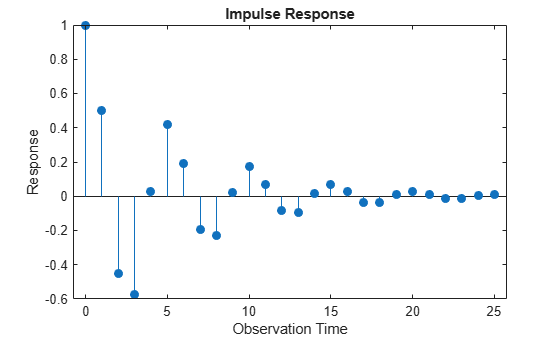# impulse

Impulse response function

## Syntax

```impulse(Mdl) impulse(Mdl,numObs) Y = impulse(___) ```

## Description

`impulse(Mdl)` plots a discrete stem plot of the impulse response function for the univariate ARIMA model, `Mdl`, in the current figure window.

`impulse(Mdl,numObs)` plots the impulse response function for `numObs` periods.

`Y = impulse(___)` returns the impulse response in a column vector for any of the previous input arguments.

## Input Arguments

 `Mdl` ARIMA model, as created by `arima` or `estimate`. `numObs` Positive integer indicating the number of observations to include in the impulse response (the number of periods for which `impulse` computes the impulse response). When you specify `numObs`, `impulse` computes the impulse response by filtering a unit impulse followed by a vector of zeros of appropriate length. The filtering algorithm is very fast and results in an impulse response of known length. If you do not specify `numObs`, `impulse` determines the number of observation using the polynomial division algorithm of the underlying lag operator polynomials, `mldivide`.

## Output Arguments

 `Y` Column vector of impulse responses. If you specify `numObs`, then `Y` is `numObs`-by-1. If you do not specify `numObs`, the underlying lag operator polynomial division algorithm returns an impulse response of generally unknown length.

## Examples

expand all

Specify the AR(2) model,

`${y}_{t}=0.5{y}_{t-1}-0.7{y}_{t-2}+{\epsilon }_{t}.$`

`Mdl = arima('AR',{0.5,-0.7},'Constant',0)`
```Mdl = arima with properties: Description: "ARIMA(2,0,0) Model (Gaussian Distribution)" Distribution: Name = "Gaussian" P: 2 D: 0 Q: 0 Constant: 0 AR: {0.5 -0.7} at lags [1 2] SAR: {} MA: {} SMA: {} Seasonality: 0 Beta: [1×0] Variance: NaN ```

Plot the impulse response function without specifying the number of observations.

`impulse(Mdl)`The model is stationary; the impulse response function decays in a sinusoidal pattern.

Specify the ARMA(1,1) model,

`${y}_{t}=0.7{y}_{t-1}+{\epsilon }_{t}+0.2{\epsilon }_{t-1}.$`

`Mdl = arima('AR',0.7,'MA',0.2,'Constant',0)`
```Mdl = arima with properties: Description: "ARIMA(1,0,1) Model (Gaussian Distribution)" Distribution: Name = "Gaussian" P: 1 D: 0 Q: 1 Constant: 0 AR: {0.7} at lag  SAR: {} MA: {0.2} at lag  SMA: {} Seasonality: 0 Beta: [1×0] Variance: NaN ```

Store the impulse response function for 15 periods.

`Y = impulse(Mdl,15)`
```Y = 15×1 1.0000 0.9000 0.6300 0.4410 0.3087 0.2161 0.1513 0.1059 0.0741 0.0519 ⋮ ```

When you specify the number of observations, you know the length of the output impulse response series.

expand all

## Tips

• To improve performance of the filtering algorithm, specify the number of observations to include in the impulse response, `numObs`. When you do not specify `numObs`, `impulse` computes the impulse response by converting the input model to a truncated, infinite-degree, moving average representation using the relatively slow lag operator polynomial division algorithm. This results in an impulse response of generally unknown length.

## References

 Box, G. E. P., G. M. Jenkins, and G. C. Reinsel. Time Series Analysis: Forecasting and Control 3rd ed. Englewood Cliffs, NJ: Prentice Hall, 1994.

 Enders, W. Applied Econometric Time Series. Hoboken, NJ: John Wiley & Sons, 1995.

 Hamilton, J. D. Time Series Analysis. Princeton, NJ: Princeton University Press, 1994.

 Lütkepohl, H. New Introduction to Multiple Time Series Analysis. New York, NY: Springer-Verlag, 2007.